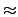Next: 48-bit CRI Linear Congruential Up: Random Number Generators Previous: 64-bit Linear Congruential Generator

## 48-bit Linear Congruential Generator

This generator is the same as the default one-stream SPRNG 48-bit lcg. This generator suffers from the same patterns in the low order bits as the 64-bit generator, but these patterns become more significant because of the smaller width of the random number state. Like the 64-bit generator, this generator is fast. The next value for the random number state, x[n], is determined from the current value, x[n - 1], by

 x[n] = a x[n - 1] + b   (mod248)   . (4)
The values of the multiplier, a = 44485709377909, and the addend, b = 11863279, are hardwired into the implementation and are known to produce a good random number list. The period of this random number generator is 2482.8 x 1014. The interface routines for this generator are: Rng_Type Rng48Seed( Rng_UInt32 i, Rng_UInt32 j );

Rng_Type Rng48Spawn( Rng_Type *x );

int iRng48( Rng_Type *x );

double dRng48( Rng_Type *x );

float fRng48( Rng_Type *x );

Our advice is to only use this generator to check application sensitivity to the quality of the random number stream.Next: 48-bit CRI Linear Congruential Up: Random Number Generators Previous: 64-bit Linear Congruential Generator